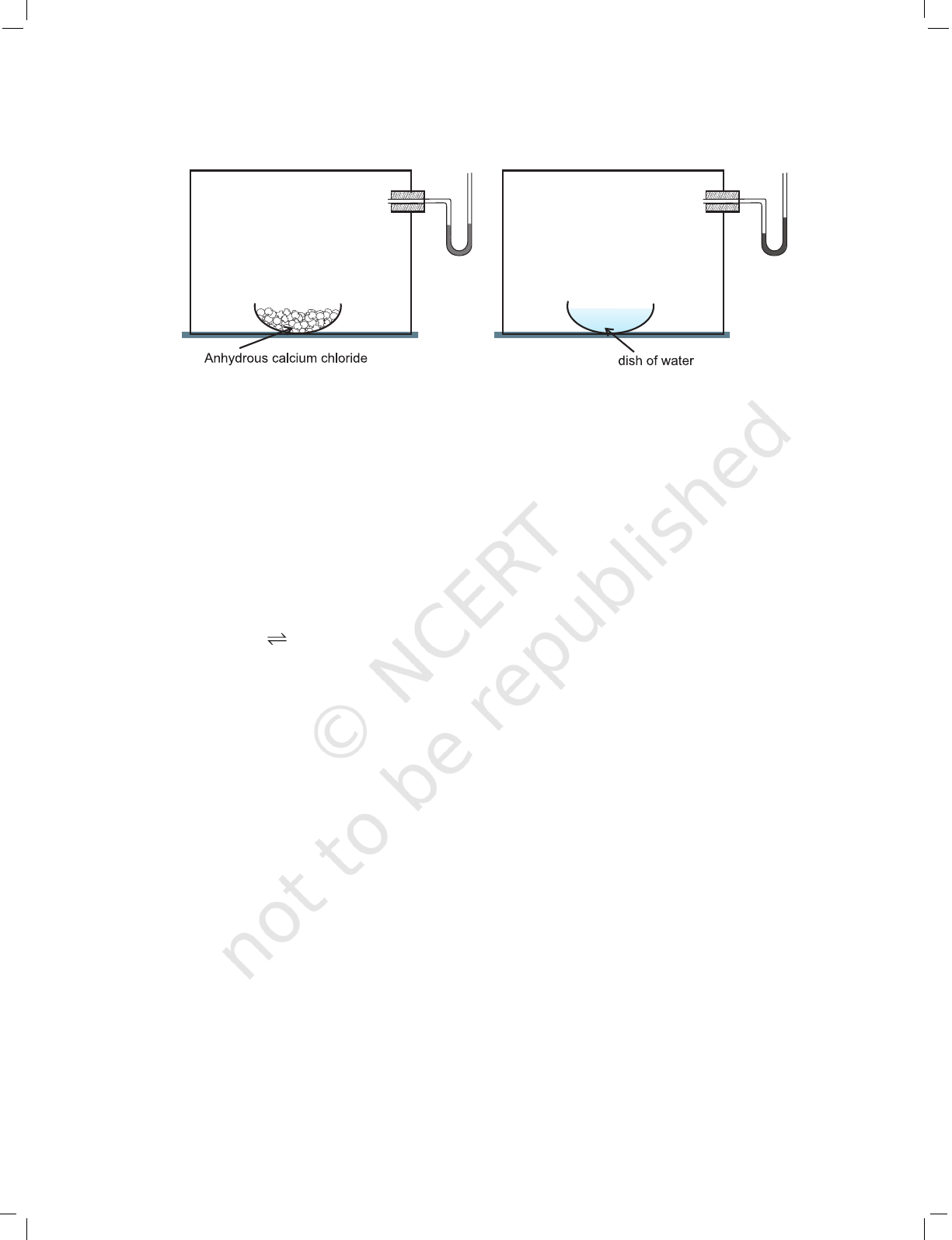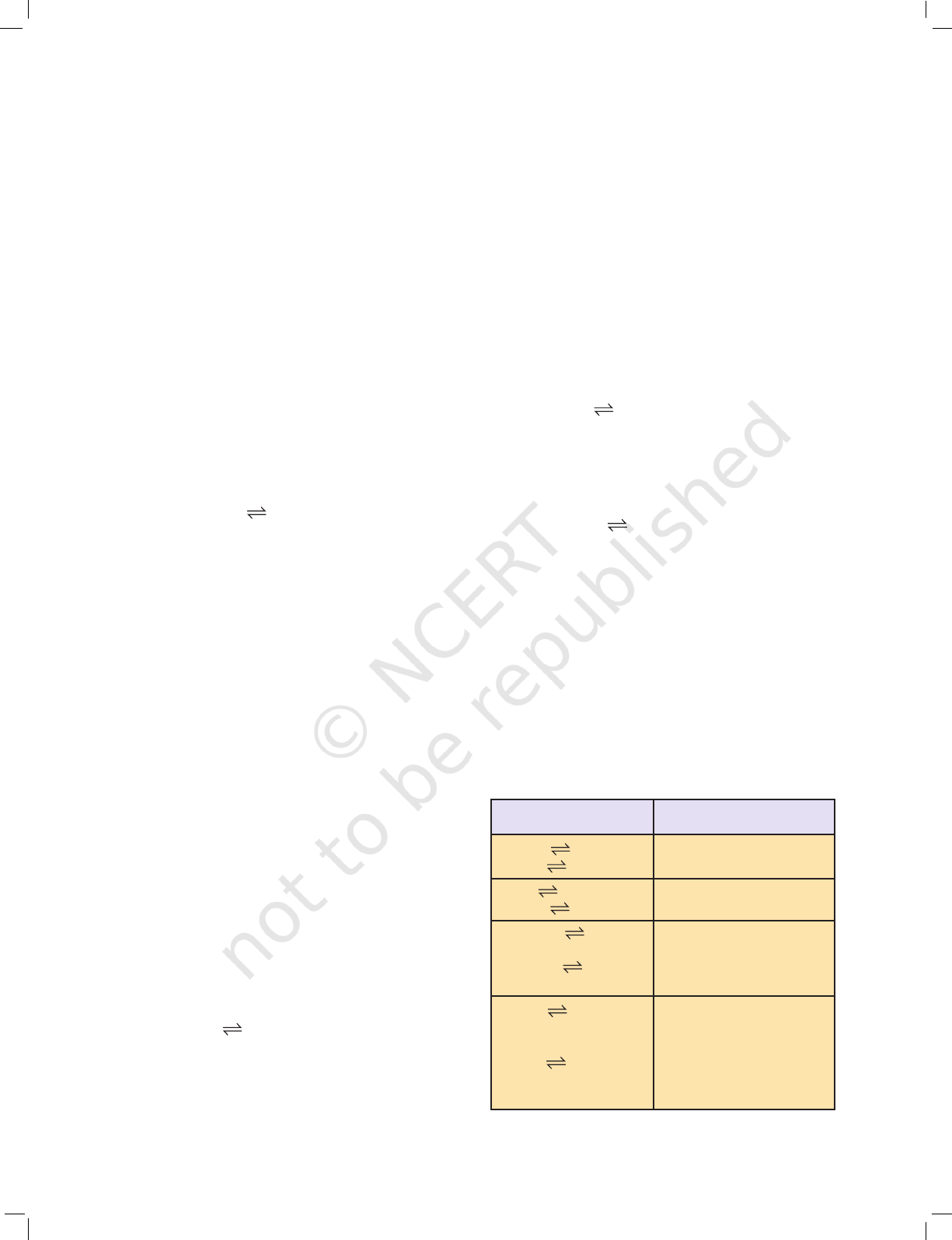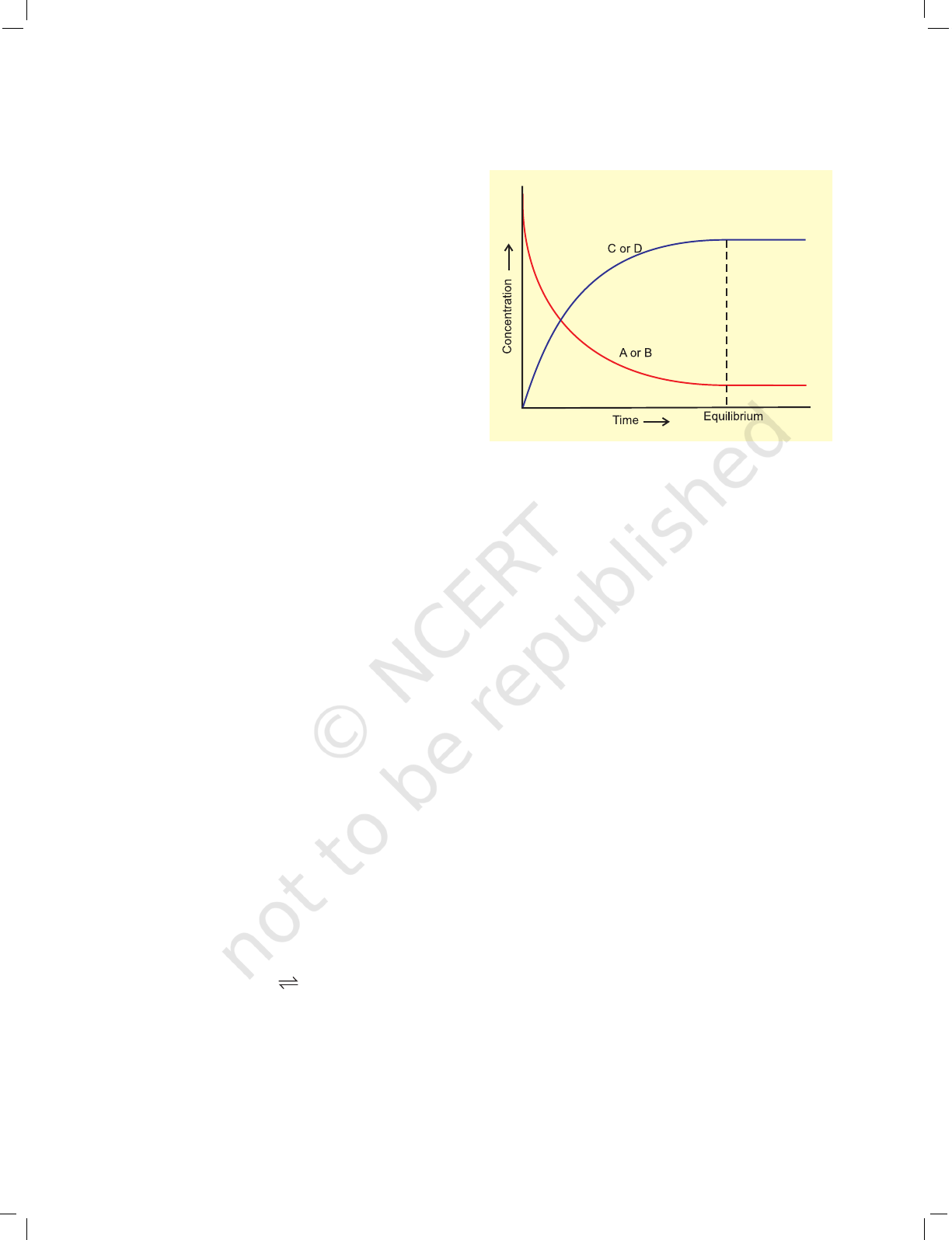192 CHEMISTRY
UNIT 7
EQUILIBRIUM
Chemical equilibria are important in numerous biological
and environmental processes. For example, equilibria
involving O
2
molecules and the protein hemoglobin play a
crucial role in the transport and delivery of O
2
from our
lungs to our muscles. Similar equilibria involving CO
molecules and hemoglobin account for the toxicity of CO.
When a liquid evaporates in a closed container,
molecules with relatively higher kinetic energy escape the
liquid surface into the vapour phase and number of liquid
molecules from the vapour phase strike the liquid surface
and are retained in the liquid phase. It gives rise to a constant
vapour pressure because of an equilibrium in which the
number of molecules leaving the liquid equals the number
returning to liquid from the vapour. We say that the system
has reached equilibrium state at this stage. However, this
is not static equilibrium and there is a lot of activity at the
boundary between the liquid and the vapour. Thus, at
equilibrium, the rate of evaporation is equal to the rate of
condensation. It may be represented by
H
2
O (l)
H
2
O (vap)
The double half arrows indicate that the processes in
both the directions are going on simultaneously. The mixture
of reactants and products in the equilibrium state is called
an equilibrium mixture.
Equilibrium can be established for both physical
processes and chemical reactions. The reaction may be fast
or slow depending on the experimental conditions and the
nature of the reactants. When the reactants in a closed vessel
at a particular temperature react to give products, the
concentrations of the reactants keep on decreasing, while
those of products keep on increasing for some time after
which there is no change in the concentrations of either of
the reactants or products. This stage of the system is the
dynamic equilibrium and the rates of the forward and
After studying this unit you will be
able to
identify dynamic nature of
equilibrium involved in physical
and chemical processes;
state the law of equilibrium;
explain characteristics of
equilibria involved in physical
and chemical processes;
write expressions for
equilibrium constants;
establish a relationship between
K
p
and K
c
;
explain various factors that
affect the equilibrium state of a
reaction;
classify substances as acids or
bases according to Arrhenius,
Bronsted-Lowry and Lewis
concepts;
classify acids and bases as weak
or strong in terms of their
ionization constants;
explain the dependence of
degree of ionization on
concentration of the electrolyte
and that of the common ion;
describe pH scale for
representing hydrogen ion
concentration;
explain ionisation of water and
its duel role as acid and base;
describe ionic product (K
w
) and
pK
w
for water;
appreciate use of buffer
solutions;
calculate solubility product
constant.
2020-21193EQUILIBRIUM
reverse reactions become equal. It is due to
this dynamic equilibrium stage that there is
no change in the concentrations of various
species in the reaction mixture. Based on the
extent to which the reactions proceed to reach
the state of chemical equilibrium, these may
be classified in three groups.
(i) The reactions that proceed nearly to
completion and only negligible
concentrations of the reactants are left. In
some cases, it may not be even possible to
detect these experimentally.
(ii) The reactions in which only small amounts
of products are formed and most of the
reactants remain unchanged at
equilibrium stage.
(iii) The reactions in which the concentrations
of the reactants and products are
comparable, when the system is in
equilibrium.
The extent of a reaction in equilibrium
varies with the experimental conditions such
as concentrations of reactants, temperature,
etc. Optimisation of the operational conditions
is very important in industry and laboratory
so that equilibrium is favorable in the
direction of the desired product. Some
important aspects of equilibrium involving
physical and chemical processes are dealt in
this unit along with the equilibrium involving
ions in aqueous solutions which is called as
ionic equilibrium.
7.1 EQUILIBRIUM IN PHYSICAL
PROCESSES
The characteristics of system at equilibrium
are better understood if we examine some
physical processes. The most familiar
examples are phase transformation
processes, e.g.,
solid
liquid
liquid gas
solid gas
7.1.1 Solid-Liquid Equilibrium
Ice and water kept in a perfectly insulated
thermos flask (no exchange of heat between
its contents and the surroundings) at 273K
and the atmospheric pressure are in
equilibrium state and the system shows
interesting characteristic features. We observe
that the mass of ice and water do not change
with time and the temperature remains
constant. However, the equilibrium is not
static. The intense activity can be noticed at
the boundary between ice and water.
Molecules from the liquid water collide against
ice and adhere to it and some molecules of ice
escape into liquid phase. There is no change
of mass of ice and water, as the rates of transfer
of molecules from ice into water and of reverse
transfer from water into ice are equal at
atmospheric pressure and 273 K.
It is obvious that ice and water are in
equilibrium only at particular temperature
and pressure. For any pure substance at
atmospheric pressure, the temperature at
which the solid and liquid phases are at
equilibrium is called the normal melting point
or normal freezing point of the substance.
The system here is in dynamic equilibrium and
we can infer the following:
(i) Both the opposing processes occur
simultaneously.
(ii) Both the processes occur at the same rate
so that the amount of ice and water
remains constant.
7.1.2 Liquid-Vapour Equilibrium
This equilibrium can be better understood if
we consider the example of a transparent box
carrying a U-tube with mercury (manometer).
Drying agent like anhydrous calcium chloride
(or phosphorus penta-oxide) is placed for a
few hours in the box. After removing the
drying agent by tilting the box on one side, a
watch glass (or petri dish) containing water is
quickly placed inside the box. It will be
observed that the mercury level in the right
limb of the manometer slowly increases and
finally attains a constant value, that is, the
pressure inside the box increases and reaches
a constant value. Also the volume of water in
the watch glass decreases (Fig. 7.1). Initially
there was no water vapour (or very less) inside
the box. As water evaporated the pressure in
the box increased due to addition of water
2020-21194 CHEMISTRY
molecules into the gaseous phase inside the
box. The rate of evaporation is constant.
However, the rate of increase in pressure
decreases with time due to condensation of
vapour into water. Finally it leads to an
equilibrium condition when there is no net
evaporation. This implies that the number of
water molecules from the gaseous state into
the liquid state also increases till the
equilibrium is attained i.e.,
rate of evaporation= rate of condensation
H
2
O(l) H
2
O (vap)
At equilibrium the pressure exerted by the
water molecules at a given temperature
remains constant and is called the equilibrium
vapour pressure of water (or just vapour
pressure of water); vapour pressure of water
increases with temperature. If the above
experiment is repeated with methyl alcohol,
acetone and ether, it is observed that different
liquids have different equilibrium vapour
pressures at the same temperature, and the
liquid which has a higher vapour pressure is
more volatile and has a lower boiling point.
If we expose three watch glasses
containing separately 1mL each of acetone,
ethyl alcohol, and water to atmosphere and
repeat the experiment with different volumes
of the liquids in a warmer room, it is observed
that in all such cases the liquid eventually
disappears and the time taken for complete
evaporation depends on (i) the nature of the
liquid, (ii) the amount of the liquid and (iii) the
temperature. When the watch glass is open to
the atmosphere, the rate of evaporation
remains constant but the molecules are
dispersed into large volume of the room. As a
consequence the rate of condensation from
vapour to liquid state is much less than the
rate of evaporation. These are open systems
and it is not possible to reach equilibrium in
an open system.
Water and water vapour are in equilibrium
position at atmospheric pressure (1.013 bar)
and at 100°C in a closed vessel. The boiling
point of water is 100°C at 1.013 bar pressure.
For any pure liquid at one atmospheric
pressure (1.013 bar), the temperature at
which the liquid and vapours are at
equilibrium is called normal boiling point of
the liquid. Boiling point of the liquid depends
on the atmospheric pressure. It depends on
the altitude of the place; at high altitude the
boiling point decreases.
7.1.3 Solid – Vapour Equilibrium
Let us now consider the systems where solids
sublime to vapour phase. If we place solid iodine
in a closed vessel, after sometime the vessel gets
filled up with violet vapour and the intensity of
colour increases with time. After certain time the
intensity of colour becomes constant and at this
stage equilibrium is attained. Hence solid iodine
sublimes to give iodine vapour and the iodine
vapour condenses to give solid iodine. The
equilibrium can be represented as,
I
2
(solid)
I
2
(vapour)
Other examples showing this kind of
equilibrium are,
Camphor (solid)
Camphor (vapour)
NH
4
Cl (solid)
NH
4
Cl (vapour)
Fig.7.1 Measuring equilibrium vapour pressure of water at a constant temperature
2020-21195EQUILIBRIUM
7.1.4 Equilibrium Involving Dissolution of
Solid or Gases in Liquids
Solids in liquids
We know from our experience that we can
dissolve only a limited amount of salt or sugar
in a given amount of water at room
temperature. If we make a thick sugar syrup
solution by dissolving sugar at a higher
temperature, sugar crystals separate out if we
cool the syrup to the room temperature. We
call it a saturated solution when no more of
solute can be dissolved in it at a given
temperature. The concentration of the solute
in a saturated solution depends upon the
temperature. In a saturated solution, a
dynamic equilibrium exits between the solute
molecules in the solid state and in the solution:
Sugar (solution) Sugar (solid), and
the rate of dissolution of sugar = rate of
crystallisation of sugar.
Equality of the two rates and dynamic
nature of equilibrium has been confirmed with
the help of radioactive sugar. If we drop some
radioactive sugar into saturated solution of
non-radioactive sugar, then after some time
radioactivity is observed both in the solution
and in the solid sugar. Initially there were no
radioactive sugar molecules in the solution
but due to dynamic nature of equilibrium,
there is exchange between the radioactive and
two phases. The ratio of the radioactive to non-
radioactive molecules in the solution increases
till it attains a constant value.
Gases in liquids
When a soda water bottle is opened, some of
the carbon dioxide gas dissolved in it fizzes
out rapidly. The phenomenon arises due to
difference in solubility of carbon dioxide at
different pressures. There is equilibrium
between the molecules in the gaseous state
and the molecules dissolved in the liquid
under pressure i.e.,
CO
2
(gas)
CO
2
(in solution)
This equilibrium is governed by Henry’s
law, which states that the mass of a gas
dissolved in a given mass of a solvent at
any temperature is proportional to the
pressure of the gas above the solvent. This
amount decreases with increase of
temperature. The soda water bottle is sealed
under pressure of gas when its solubility in
water is high. As soon as the bottle is opened,
some of the dissolved carbon dioxide gas
escapes to reach a new equilibrium condition
required for the lower pressure, namely its
partial pressure in the atmosphere. This is how
the soda water in bottle when left open to the
air for some time, turns ‘flat’. It can be
generalised that:
(i) For solid liquid equilibrium, there is
only one temperature (melting point) at
1 atm (1.013 bar) at which the two phases
can coexist. If there is no exchange of heat
with the surroundings, the mass of the two
phases remains constant.
(ii) For liquid vapour equilibrium, the
vapour pressure is constant at a given
temperature.
(iii) For dissolution of solids in liquids, the
solubility is constant at a given
temperature.
(iv) For dissolution of gases in liquids, the
concentration of a gas in liquid is
proportional to the pressure
(concentration) of the gas over the liquid.
These observations are summarised in
Table 7.1
Liquid Vapour p
H
2
O
constant at given
H
2
O (l) H
2
O (g) temperature
Solid Liquid Melting point is fixed at
H
2
O (s) H
2
O (l) constant pressure
Solute(s) Solute Concentration of solute
(solution) in solution is constant
Sugar(s) Sugar at a given temperature
(solution)
Gas(g) Gas (aq) [gas(aq)]/[gas(g)] is
constant at a given
temperature
CO
2
(g) CO
2
(aq) [CO
2
(aq)]/[CO
2
(g)] is
constant at a given
temperature
Table 7.1 Some Features of Physical
Equilibria
Process Conclusion
2020-21196 CHEMISTRY
7.1.5 General Characteristics of Equilibria
Involving Physical Processes
For the physical processes discussed above,
following characteristics are common to the
system at equilibrium:
(i) Equilibrium is possible only in a closed
system at a given temperature.
(ii) Both the opposing processes occur at the
same rate and there is a dynamic but
stable condition.
(iii) All measurable properties of the system
remain constant.
(iv) When equilibrium is attained for a physical
process, it is characterised by constant
value of one of its parameters at a given
temperature. Table 7.1 lists such
quantities.
(v) The magnitude of such quantities at any
stage indicates the extent to which the
physical process has proceeded before
reaching equilibrium.
7.2 EQUILIBRIUM IN CHEMICAL
PROCESSES – DYNAMIC
EQUILIBRIUM
Analogous to the physical systems chemical
reactions also attain a state of equilibrium.
These reactions can occur both in forward
and backward directions. When the rates of
the forward and reverse reactions become
equal, the concentrations of the reactants
and the products remain constant. This is
the stage of chemical equilibrium. This
equilibrium is dynamic in nature as it
consists of a forward reaction in which the
reactants give product(s) and reverse
reaction in which product(s) gives the
original reactants.
For a better comprehension, let us
consider a general case of a reversible reaction,
A + B
C + D
With passage of time, there is
accumulation of the products C and D and
depletion of the reactants A and B (Fig. 7.2).
This leads to a decrease in the rate of forward
reaction and an increase in he rate of the
reverse reaction,
Fig. 7.2 Attainment of chemical equilibrium.
Eventually, the two reactions occur at the
same rate and the system reaches a state of
equilibrium.
Similarly, the reaction can reach the state of
that is, no A and B being present initially, as the
equilibrium can be reached from either direction.
The dynamic nature of chemical
equilibrium can be demonstrated in the
synthesis of ammonia by Haber’s process. In
a series of experiments, Haber started with
known amounts of dinitrogen and dihydrogen
maintained at high temperature and pressure
and at regular intervals determined the
amount of ammonia present. He was
successful in determining also the
concentration of unreacted dihydrogen and
dinitrogen. Fig. 7.4 (page 191) shows that after
a certain time the composition of the mixture
remains the same even though some of the
reactants are still present. This constancy in
composition indicates that the reaction has
reached equilibrium. In order to understand
the dynamic nature of the reaction, synthesis
of ammonia is carried out with exactly the
same starting conditions (of partial pressure
and temperature) but using D
2
(deuterium)
in place of H
2
. The reaction mixtures starting
either with H
2
or D
2
reach equilibrium with
the same composition, except that D
2
and ND
3